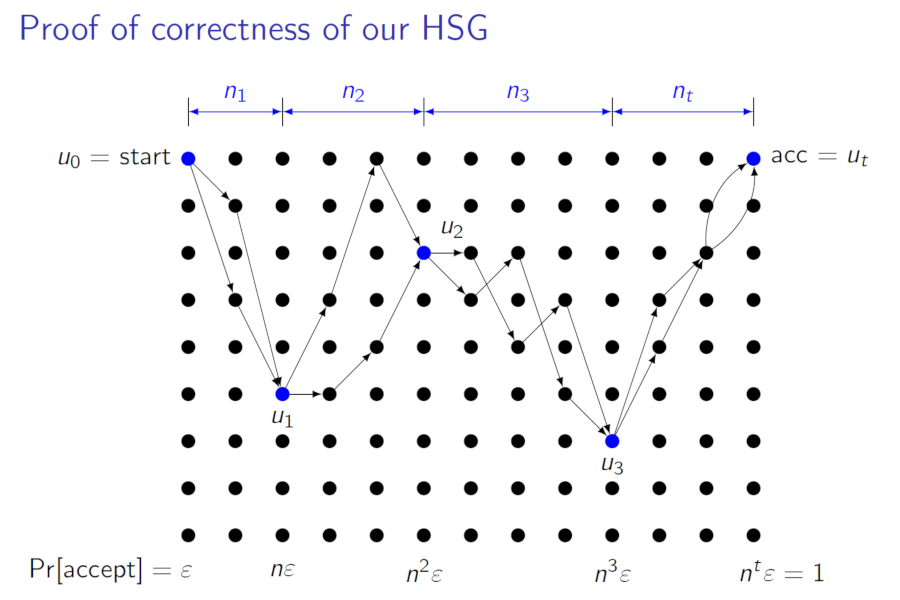Back to list of papers

# Simple Optimal Hitting Sets for Small-Success RL

By William M. Hoza and David Zuckerman

Abstract (for specialists)

We give a simple explicit hitting set generator for read-once branching programs of width $$w$$ and length $$r$$ with known variable order and acceptance probability at least $$\varepsilon$$. When $$r = w$$, our generator has seed length $$O(\log^2 r + \log(1/\varepsilon))$$. When $$r = \text{polylog } w$$, our generator has optimal seed length $$O(\log w + \log(1/\varepsilon))$$. For intermediate values of $$r$$, our generator's seed length smoothly interpolates between these two extremes. Our generator's seed length improves on recent work by Braverman, Cohen, and Garg (SICOMP 2020). In addition, our generator and its analysis are dramatically simpler than the work by Braverman et al. When $$\varepsilon$$ is small, our generator's seed length improves on all the classic generators for space-bounded computation (Nisan Combinatorica 1992; Impagliazzo, Nisan, and Wigderson STOC 1994; Nisan and Zuckerman JCSS 1996). However, all of these other works construct more general objects than we do. As a corollary of our construction, we show that every $$\mathbf{RL}$$ algorithm that uses $$r$$ random bits can be simulated by an $$\mathbf{NL}$$ algorithm that uses only $$O(r/ \log^c n)$$ nondeterministic bits, where c is an arbitrarily large constant. Finally, we show that any $$\mathbf{RL}$$ algorithm with small success probability $$\varepsilon$$ can be simulated deterministically in space $$O(\log^{3/2} n + \log n \log \log(1/\varepsilon))$$. This space bound improves on work by Saks and Zhou (JCSS 1999), who gave an algorithm for the more general "two-sided" problem that runs in space $$O(\log^{3/2} n + \sqrt{\log n} \log(1/\varepsilon ))$$.

Not-so-abstract (for curious outsiders)

⚠️ This summary might gloss over some important details.

In this paper, we present a set of binary strings $$H$$ with the following guarantee. Suppose $$P$$ is a property of binary strings such that a decent fraction of all binary strings have property $$P$$. Suppose also that there is some algorithm for determining whether a given string $$x$$ has property $$P$$, using a small amount of memory and reading $$x$$ just once from left to right. Then some string in $$H$$ has property $$P$$. Our set $$H$$ is smaller than any such set discovered previously.

We posted a manuscript online in April 2018; I presented the preliminary version of the paper at FOCS in October 2018; the paper was published in SICOMP in July 2020. The SICOMP version (pdf) has improved presentation and updated references compared to the FOCS proceedings version, which in turn has a couple extra references compared to the ECCC version. The three versions also have different formatting.

Expository material:Slides from my presentation at FOCS (October 2018). See also these slides from my longer presentation at Dagstuhl Seminar 18391 (September 2018); I used very similar slides for my presentation at China Theory Week (September 2018) and my presentation at the UT Austin CS Theory Seminar (February 2019).

🎓

An exposition of the paper is included in my PhD dissertation (Sections 3.1 and 3.2).

Video from David's presentation at Princeton Theory Lunch (October 2018).

Sumegha Garg discusses the paper in her portion of this STOC workshop (June 2020); her presentation starts at around 1:40:15.

Omer Reingold discusses the paper in this presentation for the DIMACS Day of Complexity Tutorials (July 2019).

👨‍🏫

The main result of the paper is covered in these lecture notes for a course taught by Amnon Ta-Shma (Spring 2018).